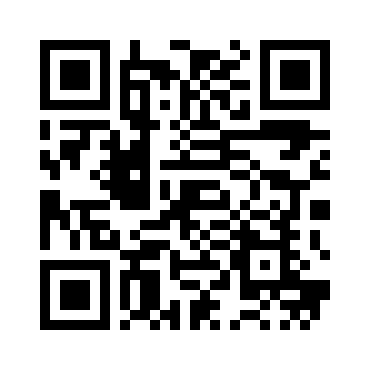# picoCTF 2019 - Java Script Kiddie 2

Posted on October 12, 2019* in ctf-writeups

We are given a website, that is nearly identical to Java Script Kiddie 1. The `assemble_png` function takes in a key of length 32, and manipulates the bytes to decode the `src` attribute of an image.

``````function assemble_png(u_in){
var LEN = 16;
var key = "00000000000000000000000000000000";
var shifter;
if(u_in.length == key.length){
key = u_in;
}
var result = [];
for(var i = 0; i < LEN; i++){
shifter = Number(key.slice((i*2),(i*2)+1));
for(var j = 0; j < (bytes.length / LEN); j ++){
result[(j * LEN) + i] = bytes[(((j + shifter) * LEN) % bytes.length) + i]
}
}
while(result[result.length-1] == 0){
result = result.slice(0,result.length-1);
}
document.getElementById("Area").src = "data:image/png;base64," + btoa(String.fromCharCode.apply(null, new Uint8Array(result)));
return false;
}
``````

Based on testing, the `document.getElementById("Area").src` will only change by 1 or 2 characters based on the input key, and it only affects the output in matching positions. That is, a key starting with `5871` will always start with `data:image/png;base64,iV`. Base64 encoded pngs always start with the following header `data:image/png;base64,iVBORw0KGgoAAAANSUhEU`. Therefore, we can easily bruteforce this. I used the following script (by pasting into Chrome DevTools Console). However, because this script only expects a change in `src` by a single character for every two input digits, this occasionally requires manual intervention by updating `cur_known` and `cur_expected`, preventing a raw bruteforce.

``````const expected_final = 'data:image/png;base64,iVBORw0KGgoAAAANSUhEU'

//let cur_known = ''
//let cur_expected = 'data:image/png;base64,i'

//let cur_known = '50706000107050002115'
//let cur_expected = 'data:image/png;base64,iVBORw0KGgoA'

//let cur_known = '507060001070500060100090'
//let cur_expected = 'data:image/png;base64,iVBORw0KGgoAAAANS'

let cur_known = '507060001070500060100090300706'
let cur_expected = 'data:image/png;base64,iVBORw0KGgoAAAANSUhEU'

ignored = new Set()

while (cur_known.length <= 32){
let found = false;
console.log(cur_known)

let cur;

outer:
for(let j = 0; j < 10; j++) {
for(let i = 0; i < 10; i++) {
cur = cur_known + j + i;

if(!ignored.has(cur)){

if(document.getElementById("Area").src.startsWith(cur_expected)) {
found = true;
break outer;
}
}
}
}

if(!found){
console.log('Backtracking', cur_known, document.getElementById("Area").src, cur_expected)
// Ignore current string
console.log(ignored)

// Backtrack - last one was wrong
cur_known = cur_known.substring(0, cur_known.length - 2);

cur_expected = cur_expected.substring(0, cur_expected.length - 1);
}

if(found){
if(cur_expected == expected_final){
break;
}
console.log('Found', cur, document.getElementById("Area").src, cur_expected)
cur_known = cur;

cur_expected = expected_final.substr(0, cur_expected.length + 1);
}
}
``````

After running this script, we are able to see the decoded image:Decoding the QR code gives us the flag:

``````picoCTF{b19be0d3b70ffc63b6367ecf136e853e}
``````DarkLight